# Analyzing 2-factor 2-level Design as if It Were 4 Different Treatment Design

Six Sigma – iSixSigma Forums General Forums Tools & Templates Analyzing 2-factor 2-level Design as if It Were 4 Different Treatment Design

Viewing 8 posts - 1 through 8 (of 8 total)
• Author
Posts
• #252449Gustav
Participant

Hello!

Just curious why we see different results in such cases.

Here we have a classic 2-factor 2-level factorial design which we can analyze using Nominal regression/logistic regression.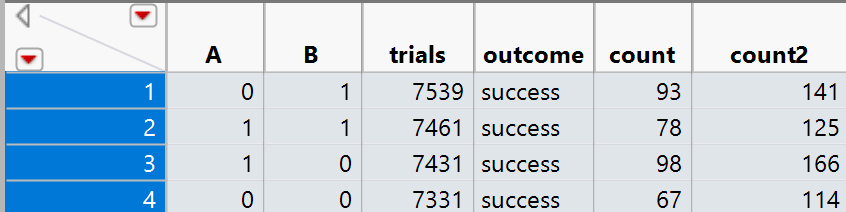Analysis shows that only interaction is significant :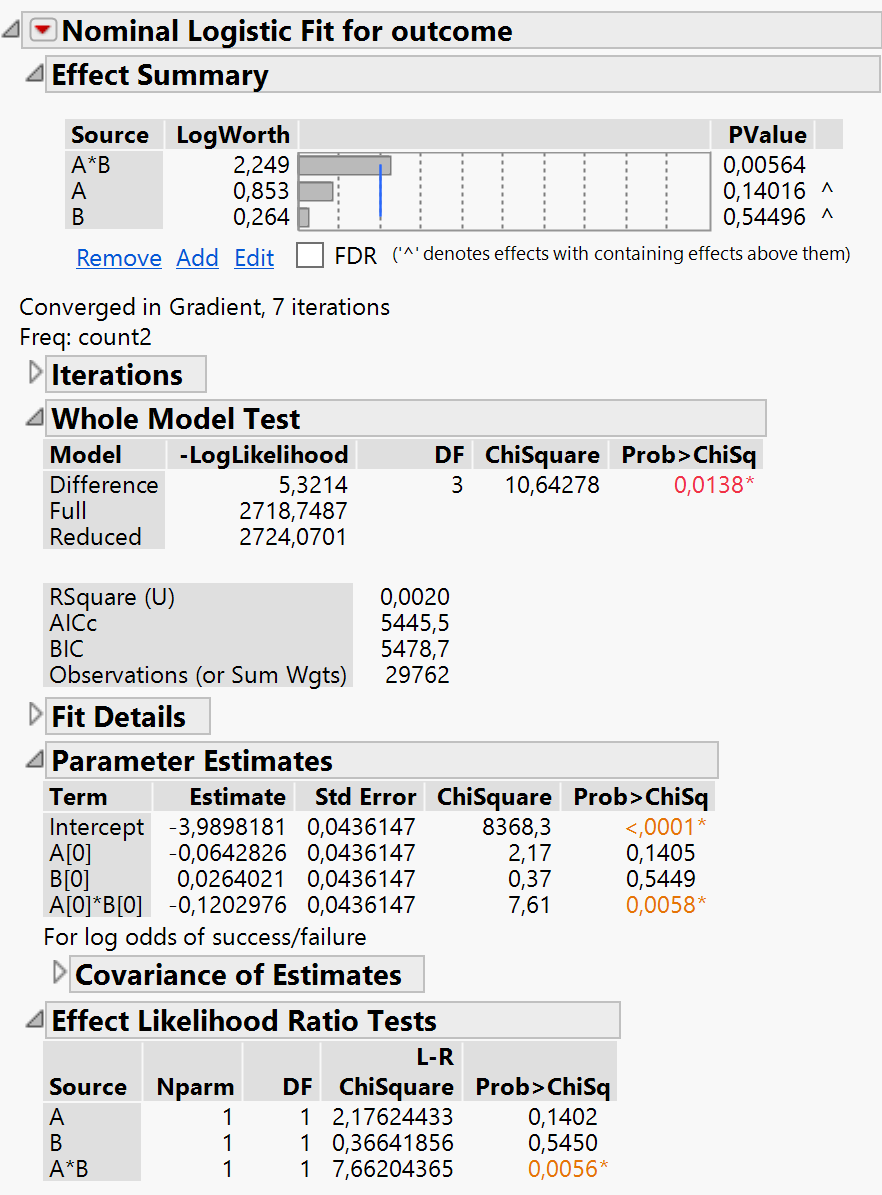However, if we transform this data as if there were 4 factors A1, B1, C (A1*B1), D (A0*B0),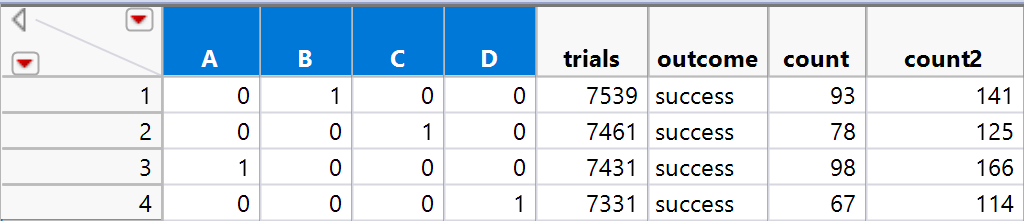then we will see that main effects are significant.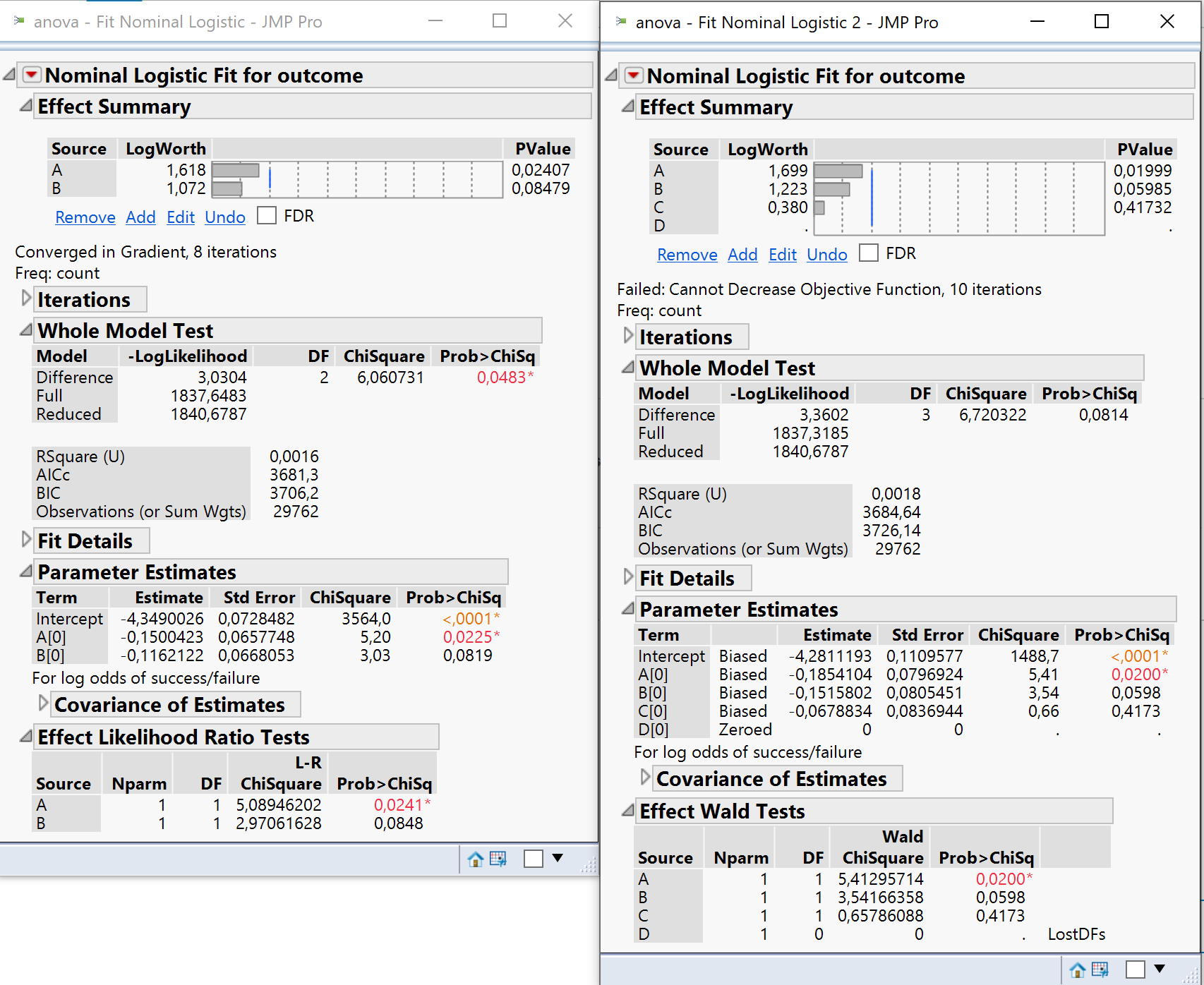How can we explain and interpret this?

PS
Datasets are in attachments. These results are from real experiment.

###### Attachments:
1. 2_4_factors.xlsx
0
#252453Gustav
Participant

Actually it is better to transform it to 1-factor 4-level design.

The results in this case show that A factor is significant.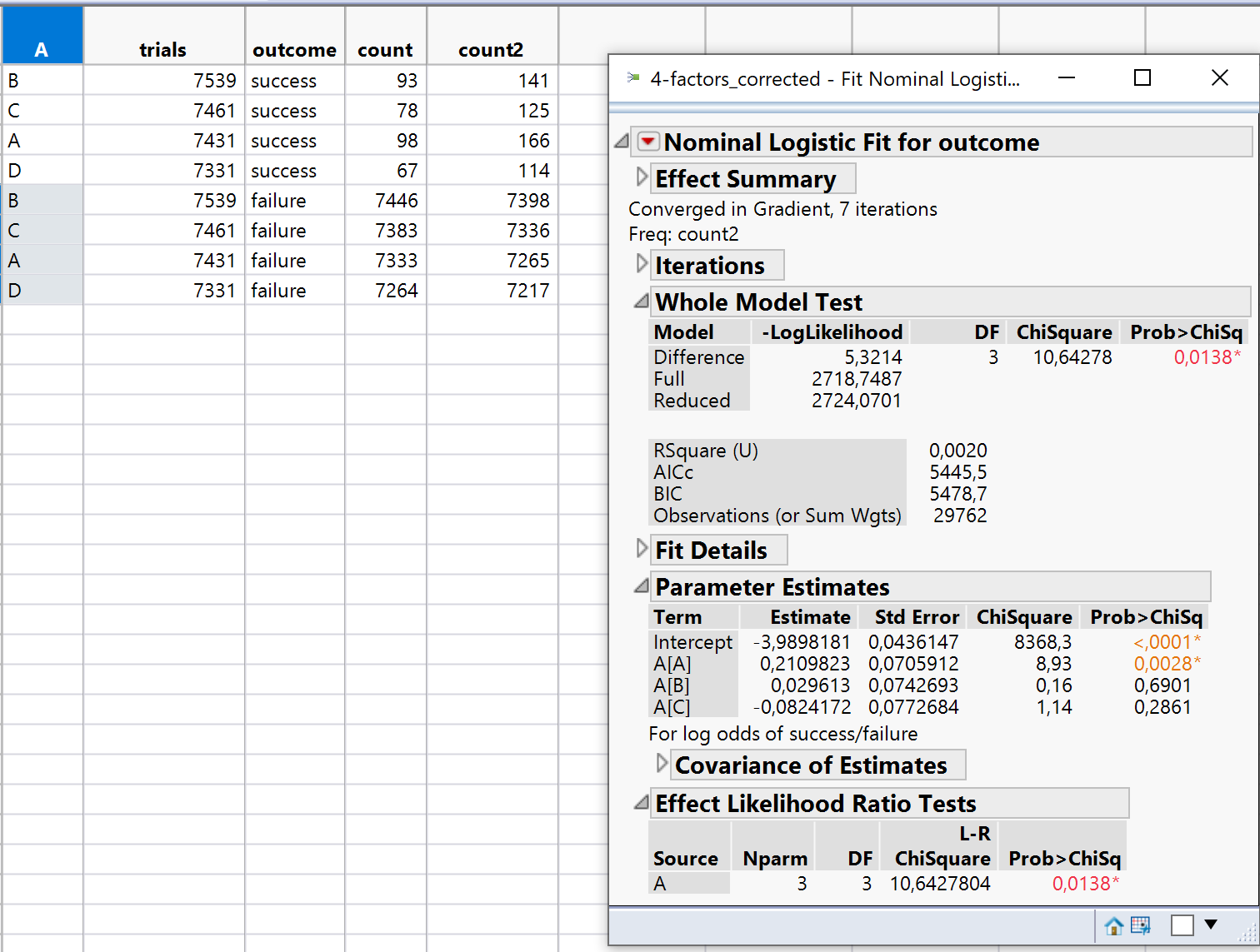0
#252463Robert Butler
Participant

To your first modification of the 2**2 factorial design.  What you said you were doing and what you did are two entirely different things.  If you are going to set D = (0,0) and C = (1,1)  (my error – C and D should be reversed but it doesn’t make a difference) where the combinations are for A and B for those particular experiments then the actual design is: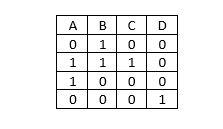What you have done is this: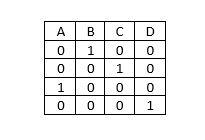This is just a standard matrix description of a one-variable-at-a-time analysis.  The fact that only the main effects are significant is not surprising – this design cannot check for interactions – indeed, this design can’t check for the 4 factors because you have 0 degrees of freedom for an error term.

Of bigger concern is you choice of regression analysis.  You said, “we can analyze [this data] using Nominal regression/logistic regression.”  In order for this to be true you would have had to run each one of those 4 experimental combinations in excess of 7000 times each because one of the key requirements of ordinary logistic regression is independence of measurements.

My guess is you ran the experiment once (or maybe twice given that you have a count and a count2 column) and then sampled each one of the runs in excess of 7000 times and determined success/failure on that basis.  This kind of data is repeated measures data and you will have to use repeated measures logistic regression methods for the analysis.

If you don’t have repeated measures capability then the only way around this is to compute percent success – if you do this with just count or count 2 then you cannot check for AXB because you only have 4 experiments.  If count and count2 are the results of a run and an actual replicate then you can put the two together – when you do – nothing is significant.  Given the percent values this isn’t surprising.

0
#252475Robert Butler
Participant

I was looking at your data set again this evening and it occurred to me if you look at the data for count and count2 there is major difference between the two columns.  If count and count2 represent the results of a design and a full replication (I doubt this since the total counts are the same for both count and count2 but bear with me) of the 2 level two variable factorial design then one could put in a third variable for replicate where -1 is the “level” corresponding to the initial run and 1 is the level for the “replicate”.  This would turn the entire experiment into a 3 variable 2 level design with a single response of count which, for the purpose of the analysis, is expressed as percent success.

Thus the design matrix would be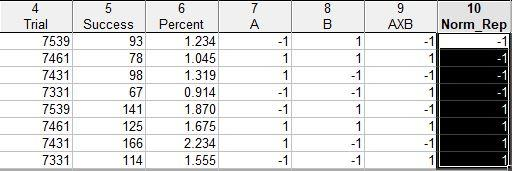If you run an analysis on this matrix where you include the terms A, B, AxB and Norm_Rep where Norm_Rep = replicate “level” then the full model is: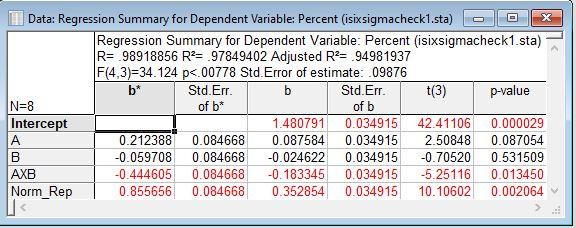Thus the variable Norm_Rep accounts for the shift between the original and replicate and when this is done the AxB interaction becomes significant.

All of the above is based on a lot of assumptions about the meaning of count and count2 and may be nothing more than playing games with numbers but I thought it was worth mentioning.

0
#252492Gustav
Participant

Number of trials is actually number of time each treatment ran, for example for A0 B1 there were 7539 runs and in 141  cases there were successes for count2 response and 93 successes for count response. I am considering now only count2 response for the analysis though.

I rewrote it in R to be a bit more clear:

A <- c(-1,+1,+1,-1)
B <- c(-1,-1,+1,+1)
trials <- c(7539,7461,7431,7331)
successes <- c(141,125,166,114)
df2 <- data.frame(A,B,trials,successes)

df2\$A <- as.factor(df2\$A)
df2\$B <- as.factor(df2\$B)

df2.glm <- glm(formula = cbind(successes, trials) ~ (A+B)^2,
family = binomial(link=logit), data = df2)

df2.glm
summary(df2.glm)

The output is:

Call:
glm(formula = cbind(successes, trials) ~ (AA + BB)^2, family = binomial(link = logit),
data = df2)

Deviance Residuals:
 0 0 0 0

Coefficients:
Estimate Std. Error z value Pr(>|z|)
(Intercept) -3.9791 0.0850 -46.813 < 2e-16 ***
AA1 -0.1100 0.1239 -0.888 0.37456
BB1 -0.1846 0.1270 -1.453 0.14616
AA1:BB1 0.4723 0.1744 2.708 0.00678 **

Signif. codes: 0 ‘***’ 0.001 ‘**’ 0.01 ‘*’ 0.05 ‘.’ 0.1 ‘ ’ 1

(Dispersion parameter for binomial family taken to be 1)

Null deviance: 1.0251e+01 on 3 degrees of freedom
Residual deviance: 2.2453e-12 on 0 degrees of freedom
AIC: 34.909

Number of Fisher Scoring iterations: 3

It shows that only interaction effect is significant which I guess is called cross-over interaction. I find it quite difficult to interpret in light of non-significance of main effects.

Since I see that AA has the biggest effects size I assume that we can declare the A1 B0 combination as one which optimizes the response count2 to the max levels.

That’s why I considered making a multiple comparison test against control using Dunnett’s test:

install.packages(multcomp)
library(multcomp)

# Dunnett’s test with multicomp

factors <- as.factor(c(“B”,”AB”,”A”,”Con”))
trials <- c(7539,7461,7431,7331)
successes <- c(141,125,166,114)

df3 <- data.frame(factors,trials,successes)

df3\$factors <- relevel(df3\$factors, ref = “Con”)

df3.glm <- glm(formula = cbind(successes, trials) ~ factors,
family = binomial(link=logit), data = df3)

dunnet1 <- glht(df3.glm,
linfct = mcp(factors = “Dunnett”),alternative = “greater”
)

summary(dunnet1)
plot(dunnet1)

The output is:

Simultaneous Tests for General Linear Hypotheses

Multiple Comparisons of Means: Dunnett Contrasts

Fit: glm(formula = cbind(successes, trials) ~ factors, family = binomial(link = logit),
data = df3)

Linear Hypotheses:
Estimate Std. Error z value Pr(>z)
A – Con <= 0 0.36224 0.12275 2.951 0.00432 **
AB – Con <= 0 0.07454 0.13055 0.571 0.49475
B – Con <= 0 0.18458 0.12702 1.453 0.16066

Signif. codes: 0 ‘***’ 0.001 ‘**’ 0.01 ‘*’ 0.05 ‘.’ 0.1 ‘ ’ 1
(Adjusted p values reported — single-step method)

Dunnett’s test shows that A1B0 is statistically significant compared to control.

These 2 methods show different results in terms of p-values and I really struggle to interpret it in the end.

0
#252512Robert Butler
Participant

No, the two methods do not show different results – the issue is you are not making the proper comparisons.

In the regression you found the AxB interaction to be significant.

In the test for group differences you chose to run a comparison against the control – this isn’t what you want to do.

There are 4 combinations that comprise the AxB interaction – A0B0, A1B0, A0B1 and A1B1.  Therefore, when testing the AxB interaction the comparisons you want to make are A0B0 vs (A1B0, A0B1, and A1B1), A1B0 vs (A0B1 and A1B1) and A0B1 vs A1B1.  When you do this you will get the following: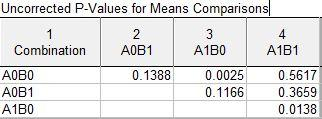My guess is if you run your analysis using a Tukey-Kramer adjustment you will probably see the A0B0 vs A1B0 and the A1B0 vs A1B1 contrasts as statistically significant.  If this isn’t the case and all that remains significant after adjustment is the A0B0 vs A1B0 comparison then that tells you the term significance is being driven by a single comparison. The situation where just one contrast is the driver for the significance of the AxB interaction is quite common.

0
#252513Gustav
Participant

@rbutler thanks again!
But why can’t I use Dunnett’s test if my purpose is to find a factor or combination of factors to optimize for max success rates?

I have done Tukey test, and it shows the same, that A factor is significant compared to Control. However,  A1B0 vs A1B1 is not.

Does it mean that in this case of logistic regression results of 2-factor 2-level experiment where we have larger effect sizes of main effects A and B (but not significant) and cross-over interaction of AB which effect size is less than of A and B but is statistically significant then we may relay on a Dunnett’s and Tukey tests?

In the end I have recommended factor A as the one who optimizes success rates maximally.

I am just doubting a bit about my conclusions.

Simultaneous Tests for General Linear Hypotheses

Multiple Comparisons of Means: Tukey Contrasts

Fit: glm(formula = cbind(successes, trials) ~ factors, family = binomial(link = logit),
data = df3)

Linear Hypotheses:
Estimate Std. Error z value Pr(>z)
A – Con <= 0 0.36224 0.12275 2.951 0.00873 **
AB – Con <= 0 0.07454 0.13055 0.571 0.75278
B – Con <= 0 0.18458 0.12702 1.453 0.28888
AB – A <= 0 -0.28770 0.11955 -2.407 1.00000
B – A <= 0 -0.17766 0.11569 -1.536 1.00000
B – AB <= 0 0.11005 0.12393 0.888 0.58412

Signif. codes: 0 ‘***’ 0.001 ‘**’ 0.01 ‘*’ 0.05 ‘.’ 0.1 ‘ ’ 1
(Adjusted p values reported — single-step method)

0
#252514Robert Butler
Participant

Because, the contrast (or contrasts) that make the AxB interaction significant may not be the set of contrasts tested by just focusing on comparisons with a control.

As for your recommendation – if your design matrix is such that the 0 setting for either A or B really means a complete absence of either A or B and not a case of A or B being at some non-zero level lower than whatever corresponds to A or B at the setting of 1 then the recommendation would be to run the combination of A1Bo which is better than A0B0 which corresponds to saying running the process with A present and B not present will be better than running the process with A and B absent – in other words running with A will be better.

On the other hand, if the matrix designation of 0 for the low level means something other than complete absence then the optimum would be running the process with A at its maximum level with B set at its minimum.

0
Viewing 8 posts - 1 through 8 (of 8 total)

You must be logged in to reply to this topic.